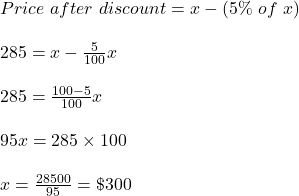## a bicycle was sold for $285 at a discount of 5% calculate the cost price of that bicycle​ Question a bicycle was sold for$285 at a discount of 5% calculate the cost price of that bicycle​

in progress 0
5 months 2021-09-05T04:11:57+00:00 2 Answers 3 views 0

## Answers ( )

Cost price = $300 Step-by-step explanation: Let the cost price be = x Discount % = 5% Price after discount =$285